# GENCHEM1-nomenclature-molecularcmpds.pptx

15 de Mar de 2023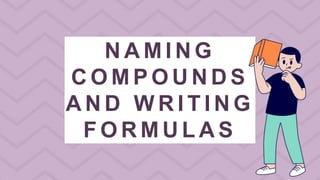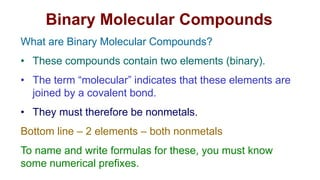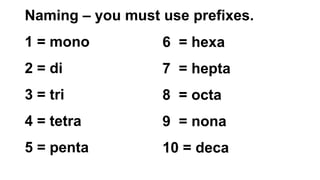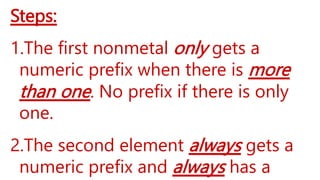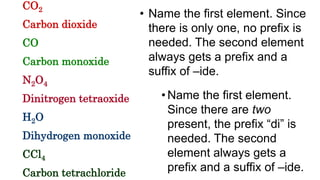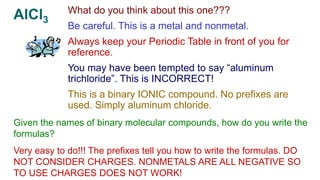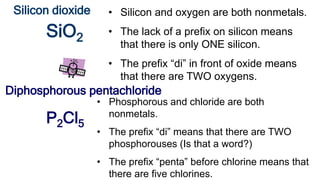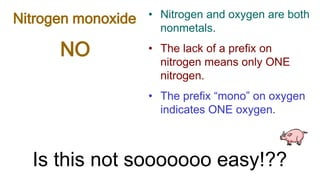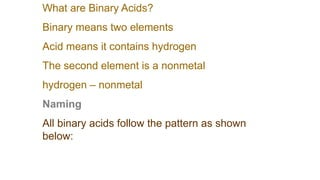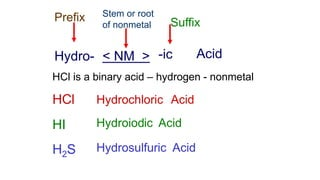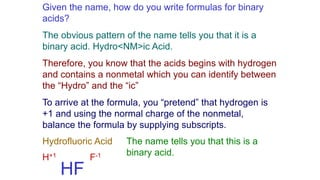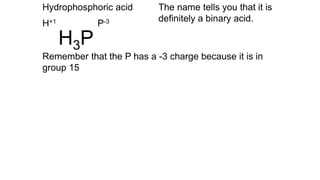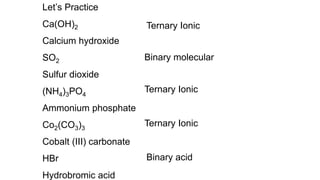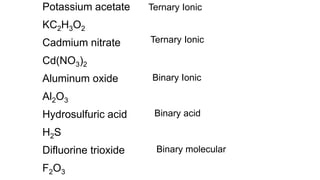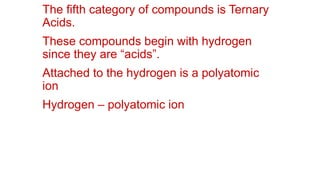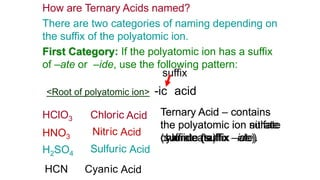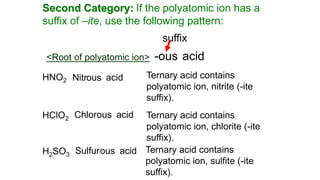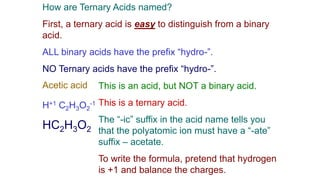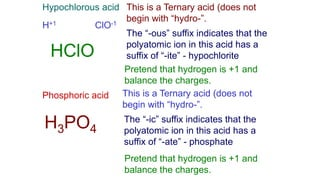1 de 20

### GENCHEM1-nomenclature-molecularcmpds.pptx

• 1. N A M I N G C O M P O U N D S A N D W R I T I N G F O R M U L A S
• 2. Binary Molecular Compounds What are Binary Molecular Compounds? • These compounds contain two elements (binary). • The term “molecular” indicates that these elements are joined by a covalent bond. • They must therefore be nonmetals. Bottom line – 2 elements – both nonmetals To name and write formulas for these, you must know some numerical prefixes.
• 3. Naming – you must use prefixes. 1 = mono 2 = di 3 = tri 4 = tetra 5 = penta 6 = hexa 7 = hepta 8 = octa 9 = nona 10 = deca
• 4. Steps: 1.The first nonmetal only gets a numeric prefix when there is more than one. No prefix if there is only one. 2.The second element always gets a numeric prefix and always has a
• 5. CO2 Carbon dioxide CO Carbon monoxide N2O4 Dinitrogen tetraoxide H2O Dihydrogen monoxide CCl4 Carbon tetrachloride • Name the first element. Since there is only one, no prefix is needed. The second element always gets a prefix and a suffix of –ide. • Name the first element. Since there are two present, the prefix “di” is needed. The second element always gets a prefix and a suffix of –ide.
• 6. AlCl3 What do you think about this one??? Be careful. This is a metal and nonmetal. Always keep your Periodic Table in front of you for reference. You may have been tempted to say “aluminum trichloride”. This is INCORRECT! This is a binary IONIC compound. No prefixes are used. Simply aluminum chloride. Given the names of binary molecular compounds, how do you write the formulas? Very easy to do!!! The prefixes tell you how to write the formulas. DO NOT CONSIDER CHARGES. NONMETALS ARE ALL NEGATIVE SO TO USE CHARGES DOES NOT WORK!
• 7. Silicon dioxide Diphosphorous pentachloride • Silicon and oxygen are both nonmetals. • The lack of a prefix on silicon means that there is only ONE silicon. • The prefix “di” in front of oxide means that there are TWO oxygens. SiO2 • Phosphorous and chloride are both nonmetals. • The prefix “di” means that there are TWO phosphorouses (Is that a word?) • The prefix “penta” before chlorine means that there are five chlorines. P2Cl5
• 8. NO Nitrogen monoxide • Nitrogen and oxygen are both nonmetals. • The lack of a prefix on nitrogen means only ONE nitrogen. • The prefix “mono” on oxygen indicates ONE oxygen. Is this not sooooooo easy!??
• 9. What are Binary Acids? Binary means two elements Acid means it contains hydrogen The second element is a nonmetal hydrogen – nonmetal Naming All binary acids follow the pattern as shown below:
• 10. Prefix Hydro- Stem or root of nonmetal < NM > Suffix -ic Acid HCl Hydro HI Hydro HCl is a binary acid – hydrogen - nonmetal chloric Acid iodic Acid H2S Hydrosulfuric Acid
• 11. Given the name, how do you write formulas for binary acids? The obvious pattern of the name tells you that it is a binary acid. Hydro<NM>ic Acid. Therefore, you know that the acids begins with hydrogen and contains a nonmetal which you can identify between the “Hydro” and the “ic” To arrive at the formula, you “pretend” that hydrogen is +1 and using the normal charge of the nonmetal, balance the formula by supplying subscripts. Hydrofluoric Acid The name tells you that this is a binary acid. H+1 F-1 HF
• 12. Hydrophosphoric acid The name tells you that it is definitely a binary acid. H+1 P-3 Remember that the P has a -3 charge because it is in group 15 H3P
• 13. Let’s Practice Ca(OH)2 Calcium hydroxide SO2 Sulfur dioxide (NH4)3PO4 Ammonium phosphate Co2(CO3)3 Cobalt (III) carbonate HBr Hydrobromic acid Ternary Ionic Binary molecular Ternary Ionic Ternary Ionic Binary acid
• 14. Potassium acetate KC2H3O2 Cadmium nitrate Cd(NO3)2 Aluminum oxide Al2O3 Hydrosulfuric acid H2S Difluorine trioxide F2O3 Ternary Ionic Ternary Ionic Binary Ionic Binary acid Binary molecular
• 15. The fifth category of compounds is Ternary Acids. These compounds begin with hydrogen since they are “acids”. Attached to the hydrogen is a polyatomic ion Hydrogen – polyatomic ion
• 16. <Root of polyatomic ion> suffix -ic acid HClO3 Chlor HNO3 Nitr H2SO4 Sulfur HCN Cyan How are Ternary Acids named? There are two categories of naming depending on the suffix of the polyatomic ion. First Category: If the polyatomic ion has a suffix of –ate or –ide, use the following pattern: Ternary Acid – contains the polyatomic ion chlorate (suffix –ate) Ternary Acid – contains the polyatomic ion nitrate (suffix –ate). Ternary Acid – contains the polyatomic ion sulfate (suffix –ate). Ternary Acid – contains the polyatomic ion cyanide (suffix –ide). ic Acid ic Acid ic Acid ic Acid
• 17. Second Category: If the polyatomic ion has a suffix of –ite, use the following pattern: <Root of polyatomic ion> suffix -ous acid HNO2 Ternary acid contains polyatomic ion, nitrite (-ite suffix). Nitrous acid HClO2 Ternary acid contains polyatomic ion, chlorite (-ite suffix). Chlorous acid H2SO3 Ternary acid contains polyatomic ion, sulfite (-ite suffix). Sulfurous acid
• 18. How are Ternary Acids named? First, a ternary acid is easy to distinguish from a binary acid. ALL binary acids have the prefix “hydro-”. NO Ternary acids have the prefix “hydro-”. Acetic acid This is an acid, but NOT a binary acid. This is a ternary acid. The “-ic” suffix in the acid name tells you that the polyatomic ion must have a “-ate” suffix – acetate. To write the formula, pretend that hydrogen is +1 and balance the charges. H+1 C2H3O2 -1 HC2H3O2
• 19. Hypochlorous acid This is a Ternary acid (does not begin with “hydro-”. The “-ous” suffix indicates that the polyatomic ion in this acid has a suffix of “-ite” - hypochlorite Pretend that hydrogen is +1 and balance the charges. H+1 ClO-1 HClO Phosphoric acid This is a Ternary acid (does not begin with “hydro-”. The “-ic” suffix indicates that the polyatomic ion in this acid has a suffix of “-ate” - phosphate H3PO4 Pretend that hydrogen is +1 and balance the charges.
• 20. Thanks! Any questions? Don't hesitate to ask for our help